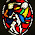Monday, 5 January 2015

Cancer risk - an analysis

My previous post discussed this paper, and its claim that two thirds of cancer types are largely unaffected by environmental or hereditary carcinogenic factors.  While I'm unimpressed by the paper, the idea behind it is interesting, so here's my analysis of its data.

The hypothesis is that "many genomic changes occur simply by chance during DNA replication rather than as a result of carcinogenic factors.  Since the endogenous mutation rate of all human cell types appears to be nearly identical, this concept predicts that there should be a strong, quantitative correlation between the lifetime number of divisions among a particular class of cells within each organ (stem cells) and the lifetime risk of cancer arising in that organ."

So let's suppose that each stem cell division gives rise to cancer with a small probability p.  Then if there are n lifetime divisions, the probability that none of them leads to cancer is (1-p)n, so the lifetime risk of cancer, R, is 1 - (1-p)n.  We can rearrange that to find an expression for p, ln(1-p) = ln(1-R)/n.  For very small p, ln(1-p) = -p, so p = -ln(1-R)/n.  If we plot ln(1-R) against n we should expect to find that for all the organs where carcinogenic factors are absent the values fall on the same straight line through the origin.

However, the values of n range through several orders of magnitude, so we can't create this plot unless we're willing to make all the rare cancers invisibly close to the origin.  Instead, let's take logs again, giving log(p) = log(-ln(1-R)) - log(n).  So on graph of log(-ln(1-R)) against log(n), all the cancers satisfying our hypothesis should fall on a straight line with slope one crossing the y axis at log(p).  (I've switched to base-10 logarithms for this step, to make the powers of ten easier to follow)

Here's the graph, which looks not unlike the one in the paper.  The correlation between the x and y data series is 0.787, again not unlike in the paper.  But the slope of a line through the points is not unity, nor is there a subset of points at the bottom of the envelope of points for which the slope is unity.

(I've arbitrarily given FAP colorectal a cancer risk of one millionth less than one, because the method doesn't allow a risk of exactly one.  Its point could be moved vertically by choosing a different number.)

To explore further how well the data fit the model, I've backed out implied values of p for each cancer type.

Here's the problem.  If the data matched the theory, there would be a group of cancer types at the left end of the chart with similar implied probabilities.  It seems in particular that the risk of small-intestine adenocarcinoma is anomalously low.

[A commentator points out that there is a group of cancer types near the left end of the chart which do have similar implied probabilities (the same eight cancers lie roughly in a straight line in the scatter plot).  But the theory in the paper is that there's a background rate of cancer in any tissue type, depending only on the number of stem cell divisions, because "the endogenous mutation rate of all human cell types appears to be nearly identical".  This theory can't be casually modified to allow for a background rate of cancer in all tissue types except for in the small intestine.  (Oncologists are of course aware that small-bowel cancers are strangely rare.)]

Let's try an alternative theory: that for every tissue type, some fraction of stem cell divisions, call it α, are affected by environmental or heriditary influences in a way which gives them a probability, call it q, of causing cancer.  q is the same for all tissue types.  The remaining divisions carry negligible risk by comparison.  Somewhat arbitrarily, we'll assume α is one for the cancer with the highest implied probability in our previous analysis: that is, q is equal to the p implied for Gallbladder non-papillary adenocarcinoma.  We can now back out a value of α for each cancer.

Well, it's a simplistic theory, but it does have the advantage over our previous model that it fits the data.

It seems to me that picking out gallbladder cancer as high-alpha is a plus for this model, because that cancer has a peculiar geographic spread which can only be due to environmental or hereditary factors.

And I've been mischievous.  In this theory, despite the correlation in the input data between stem cell divisions and cancer risk, every cancer is caused by environmental or hereditary factors.

1.You might find this interesting: http://freethoughtblogs.com/pharyngula/2015/01/05/on-the-importance-of-luck/

2.> If the data matched the theory, there would be a group of cancer types at the left end of the chart with similar implied probabilities

But there is (if you discount the very last one). The 8 before that are all very similar, just above 1e-14.

3.Your analysis is very detailed and impressive. A worth reading.
For my little and modest contribution I have followed the inverse path, building a model and generating the data according to it, so to show that a scatterplot similar to the one of the published study is compatible to the opposite hyphotesis that most cancers are due to risk factors.
http://www.antoniorinaldi.it/due-su-tre-non-per-sfortuna-ma-per-forzatura/
(to try different versions of the chart)
(to read in Machivellian English)

P.S.: google captcha is a blood bath!

4.I was more than happy to uncover this great site. I need to to thank you for your time due to this fantastic read!! I definitely enjoyed every bit of it and I have you bookmarked to see new information on your blog.
ipl auction 2017
Good Friday images
Ganesh Chaturthi images

5.Use this article to increase your knowledge . cara menggugurkan kandungan

6.By reading this article I get a lot of lessons and this is very useful .

7.